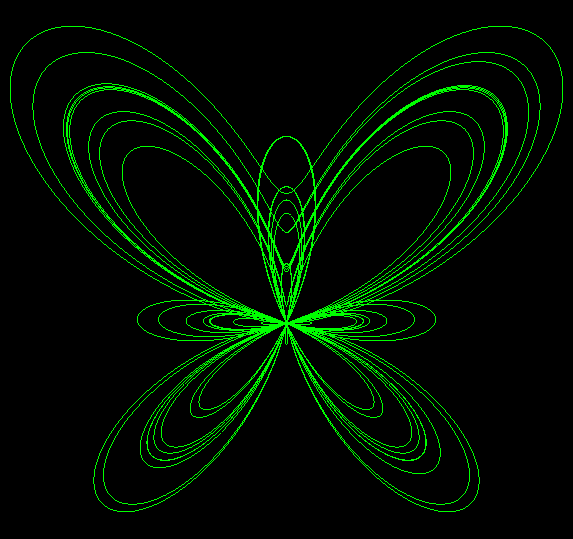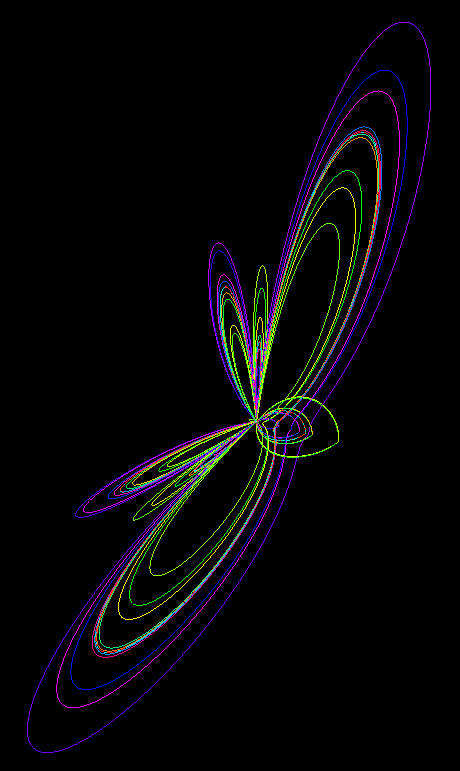# Butterfly Curve

Written by Paul Bourke

Attributed to Temple FayThe so called "butterfly" curve is given by the equation

x = cos(u) (ecos(u) - 2 cos(4 u) - sin(u / 12)5.0)
y = sin(u) (ecos(u) - 2 cos(4 u) - sin(u / 12)5.0)```#include "stdlib.h"
#include "stdio.h"
#include "math.h"

#define N 10000

typedef struct {
double x,y,z;
} XYZ;

int main(int argc,char **argv)
{
int i;
double u;
XYZ p,plast;

for (i=0;i<N;i++) {
u = i * 24.0 * PI / N;
p.x = cos(u) * (exp(cos(u)) - 2 * cos(4 * u) - pow(sin(u / 12),5.0));
p.y = sin(u) * (exp(cos(u)) - 2 * cos(4 * u) - pow(sin(u / 12),5.0));
p.z = fabs(p.y) / 2;
colour = GetColour(u,0.0,24*PI,4);
if (i > 0) {
Do something with the line from plast to p
}
plast = p;
}
}
```Anaglyph contribution by Jean Tousset.[Click for higher resolution version]

References

Temple H. Fay, "The Butterfly Curve" American Mathematical Monthly 96, No. 5, May 1989.

Temple H. Fay, "A Study in Step Size" Mathematics Magazine 70, No 2, April 1997